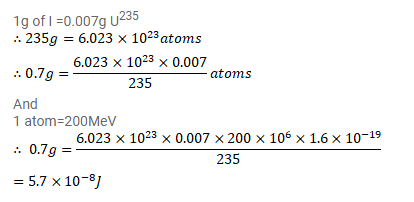# Calculate the energy released by

Question:

Calculate the energy released by $1 \mathrm{~g}$ of natural uranium assuming $200 \mathrm{MeV}$ is released in each fission event and that the fissionable isotope ${ }^{235} \mathrm{U}$ has an abundance of $0.7 \%$ by weight in natural uranium.

Solution: